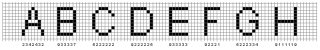# OCR

## Recommended Posts

how do I load a picture into a variable and then use pixelgetcolor to search for it?

doesn't pixelgetcolor only work for pixel on the screen？

##### Share on other sites

in example scripts there are some udfs for picture management.

One i wrote a while ago that using bitmaps- http://www.autoitscript.com/forum/index.php?showtopic=27362

probly newer ones if you look that do jpegs and such also

##### Share on other sites

work this one then.

##### Share on other sites

can you tell me very simply how to do getpixelcolor with a screenshot shot by auto3lib that's stored in a variable?

##### Share on other sites

how do I search for a image stored in the variable ?

##### Share on other sites

Ok, I figure this is the best general tool ever for potential OCR scientists.

I want to code a tool that will produce pictures like below based on your definition.

So, please lend me a hand in creating this.

How can this be acomplished?

First, a window has to be created, and then the enlargement of the area slected will be displayed.

how do I do this ?##### Share on other sites

how do i use au3 to creat image?

##### Share on other sites

is anyone interested in a jointed project for a gui for OCR ?

##### Share on other sites

Pixelgetcolor will get a point on the screen, yet, for OCR, to fit into any application, it must be able to load a picture into a variable, and then do the search there.

How can it be done, pixelcolor on a image saved in a variable?

##### Share on other sites

I do not know how to do this... thus I didn't post.

Search the forum for OCR. There have been a few made in the past for Diablo/Diablo II.

-AutoIt Smith

##### Share on other sites

```#include<array.au3>

Global \$color = 14018275
Dim \$string
Dim \$x = 0
Dim \$y = 767
Dim \$x_size = @DesktopWidth
Dim \$y_size = @DesktopHeight
Dim \$display_string = ""
Dim \$array_order = 0
Dim \$avRET

\$avRET = search_for_this_color(\$x_size, \$y_size, \$color)

MsgBox (0, "the color is at", \$avRET  )
MsgBox (0, "the color is at", \$avRET  )

\$string = ocr_string ( \$avRET)

\$matches = StringRegExp(\$string, '[^0]{1,}', 3)

_ArrayDisplay(\$matches, 'found')

Do

;check definition
If  check_definition( \$matches[\$array_order] ) == 2 Then
MsgBox ( 0, "Unknown string", \$matches[\$array_order] )
EndIf

\$display_string = \$display_string & check_definition( \$matches[\$array_order] )

\$array_order = \$array_order + 1

Until \$array_order = 2;size of the array.

MsgBox ( 0, "The string says", \$display_string )

Func search_for_this_color(\$x_size , \$y_size, \$color)
; \$avRET = X, \$avRET = Y
Local \$avRET = [0, \$y_size]
Do
Do
If PixelGetColor(\$avRET, \$avRET) = \$color Then
Return \$avRET
EndIf
\$avRET = \$avRET - 1
Until \$avRET = 0
\$avRET = \$y_size
\$avRET = \$avRET + 1
Until \$avRET = \$x_size

MsgBox (0, "title", "found nothing." )

Return SetError(1, 0, -1)
EndFunc   ;==>search_for_this_color

Func    ocr_string ( \$avRET)

Local \$x_offset = 0
Local \$y_offset = -4
Local \$box = 0

Do
Do
If PixelGetColor ( (\$avRET + \$x_offset) , (\$avRET + \$y_offset  ) ) = \$color   Then
\$box = \$box + 1
EndIf

\$y_offset = \$y_offset + 1
Until \$y_offset = 7

\$y_offset = -4
\$string = \$string &\$box
\$x_offset = \$x_offset + 1
\$box = 0

Until \$x_offset = 147                   ;this is how long this string can be, at most.

MsgBox (0, "147", \$string )
Return \$string
EndFunc

Func check_definition(  \$string )
If \$string == ""    Then
Return "-"
EndIf
If \$string == ""    Then
Return "0"
EndIf
If \$string == ""    Then
Return "1"
EndIf
If \$string == ""    Then
Return "2"
EndIf
If \$string == ""    Then
Return "3"
EndIf
If \$string == ""    Then
Return "4"
EndIf
If \$string == ""    Then
Return "5"
EndIf
If \$string == ""    Then
Return "6"
EndIf
If \$string == ""    Then
Return "7"
EndIf
If \$string == ""    Then
Return "8"
EndIf
If \$string == ""    Then
Return "9"
EndIf
If \$string == ""    Then
Return "0"
EndIf

Return 2  ;if nothing matched, return 2
EndFunc```

I haven't defined the numbers yet, what I am confused about is, the 3rd item

in the found msgbox should be "243385" but instead of that, the number is strange, and

to_search.bmp

##### Share on other sites

just open the picture in mspaint, and then run the script with the picture visible.

## Create an account

Register a new account

• ### Recently Browsing   0 members

×

• Wiki

• Back

• #### Beta

• Git
• FAQ
×
• Create New...